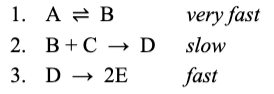# A generic reaction occurs via the following sequence of elementary steps. What is the rate law based on this reaction mechanism? A.rate =k [E]2 D.rate =k [D]. B.rate =k [B][C]. E.rate =k [A][C]. C.rate =k [A][B]